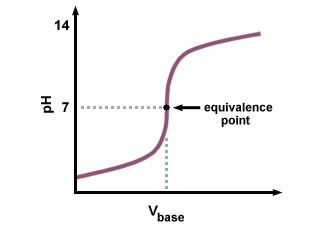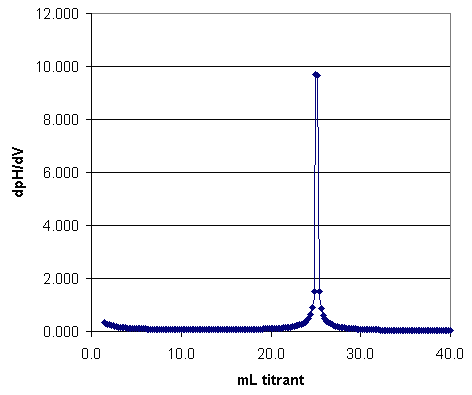HOME | INTRODUCTION | PRELAB | PROCEDURE | REPORT | PRINTABLE PDFPotentiometric Titration of an Acid Mixture : Introduction

In this experiment a mixture of two acids is titrated with standard base. Because the pH does not change abruptly enough at either equivalence point to permit estimation with visual indicators, a potentiometric titration with a pH meter and glass pH electrode-reference electrode pair is employed. A plot of pH vs. volume of base added yields a curve similar to Figure 13-2 in Skoog (11.6(a) in Harris.)Figure 1. Acid Titation with Strong Base

From the pH vs. volume curve the two end points, and thus the amount of acid present, can be found. However, a more accurate estimate of the end points can be made from a derivative plot; that is, d(pH)/d(vol) vs. volume of base added.Figure 2. Derivative Plot of Acid Titation with Strong Base

A mixture of two acids can be analyzed with a precision of a few parts per thousand, provided the dissociation constants of the two acids differ by at least 103 to 104 and the weaker acid is not so weak that its end point is completely obscured. The normality of the sodium hydroxide titrant is determined by titration of a standard acid. For highest precision the end points of the standardization and the acid being titrated are taken at the same pH; this procedure corrects for any error due to carbonate that may be present in the base. Because two end points at substantially different pH values are obtained in this experiment, calculation of two different effective concentrations for the same sodium hydroxide titrant is required.

Titration Curves

The first titration curve (of strong acid/strong base) interaction you have already seen. Use it to compare to the others.

The following titration curve interaction relates to a strong base added to a weak acid.

The last titration curve interaction relates to strong acid added to a weak base.

Click to get table summarizing of curves.